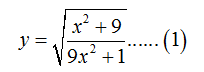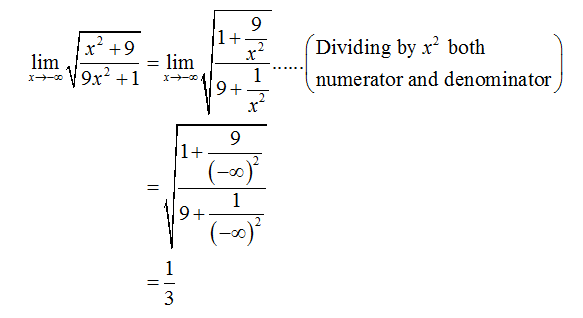# Use limits to determine the equations for all horizontal asymptote. y = (sqrt(x2+9)/(sqrt(9 x2+1))

Question
1 views

Use limits to determine the equations for all horizontal asymptote.

y = (sqrt(x2+9)/(sqrt(9 x2+1))

check_circle

Step 1

The given function isStep 2

To find the equation for horizontal asymptotes using the definition of limit.

Step 3

The horizontal asymptote for the given function can be evaluated by taking the limit of the function at positive and negative infinity. Therefore, from (1)...

### Want to see the full answer?

See Solution

#### Want to see this answer and more?

Solutions are written by subject experts who are available 24/7. Questions are typically answered within 1 hour.*

See Solution
*Response times may vary by subject and question.
Tagged in